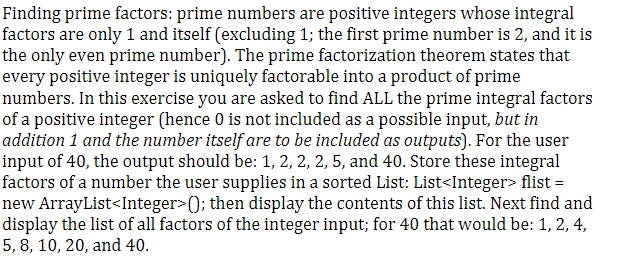# help with java code please here 39 s the assignment here 39 s the code import java u 5151822

Here&#39;s the assignment:Here&#39;s the code:

import java.util.ArrayList;
import java.util.Scanner;

public class Prime {

public static void main(String[] args) {

ArrayList factors = new ArrayList<>();
System.out.print(“Enter a whole number: “);
Scanner scanner = new Scanner(System.in);

int s = 2;

int n = scanner.nextInt();

// loop till divisors is less than dividend

while(s

// divide n by s till it is divisible

while(n !=1 && n%s==0){

n = n/s;

}

s = s+1;

}

System.out.println(factors);

// finding factors

for(int i=1; i

if(scanner.nextInt()%i == 0)

System.out.print(i+” “);

}

System.out.println();

}

}

————-

Issue:

It&#39;s making me input number twice in order to get an output, why is this happening? Can someone please help fix it

Finding prime factors: prime numbers are positive integers whose integral factors are only 1 and itself (excluding 1; the first prime number is 2, and it is the only even prime number). The prime factorization theorem states that every positive integer is uniquely factorable into a product of prime numbers. In this exercise you are asked to find ALL the prime integral factors of a positive integer (hence 0 is not included as a possible input, but in addition 1 and the number itself are to be included as outputs). For the user input of 40, the output should be: 1, 2, 2, 2,5, and 40. Store these integral factors of a number the user supplies in a sorted List: List flist = new ArrayListO; then display the contents of this list. Next find and display the list of all factors of the integer input; for 40 that would be: 1, 2, 4, 5, 8, 10, 20, and 40.